# Tic Tac Toe In C Programming With Source Code

## Tic Tac Toe In C Programming With Source Code

The Tic Tac Toe In C Programming With Source Code is developed using C programming language. This Tic Tac Toe in C game is played on a 3×3 grid by two players.

A tic tac toe game c program first player uses a circle to mark his movements, while the second uses a cross. The tic tac toe using c player who has made a three-mark horizontal, vertical, or diagonal series wins.

In this tic tac toe on c, both players must enter a particular number one to nine based on the grid position in order to make a mark X or O. The tic tac toe program in c 3×3 winner will be the first player to successfully position three of their marks in a horizontal, vertical, or diagonal) row.

Tic Tac Toe in C Programming with S...
Tic Tac Toe in C Programming with Source Code 2021 | C Project with Source Code Free Download

Anyway if you want level up your knowledge in programming especially C/C++ Programming Language, try this new article I’ve made for you Best C Projects with Source Code for Beginners Free Download 2022.

To run this tic tac toe program in c with output make sure that you have a Code Blocks or any platform of C++ installed in your computer.

## Tic Tac Toe In C Programming With Source CodeSteps On How To Run The Project

Time needed: 5 minutes.

These are the steps on how to run Tic Tac Toe In C Programming With Source Code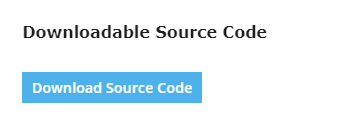• Step 2: Extract file.

Second, after you finished download the source code, extract the zip file.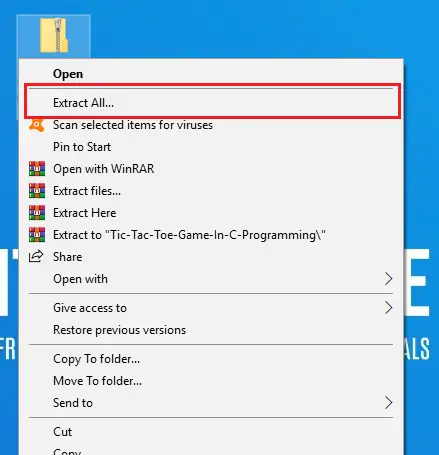• Step 3: Open CodeBlocks

Third, open “CodeBlocks IDE”.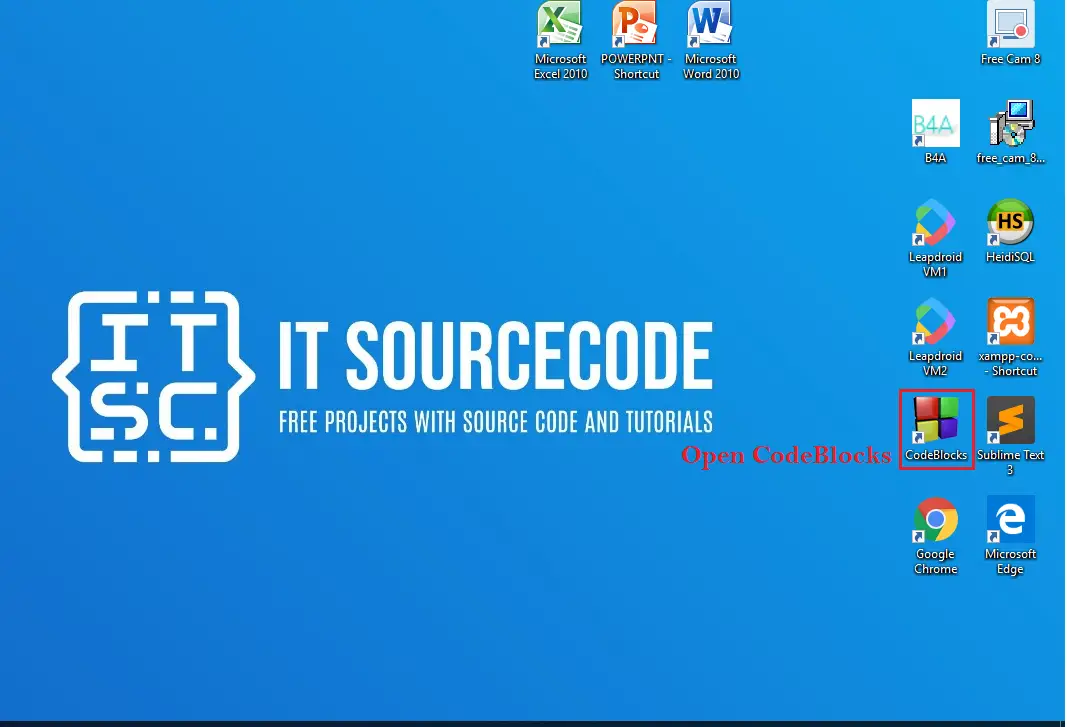• Step 4: Open Project.

Fourth, open file tab and Open File after that open folder TicTacToeC then click the “tictactoec.c“.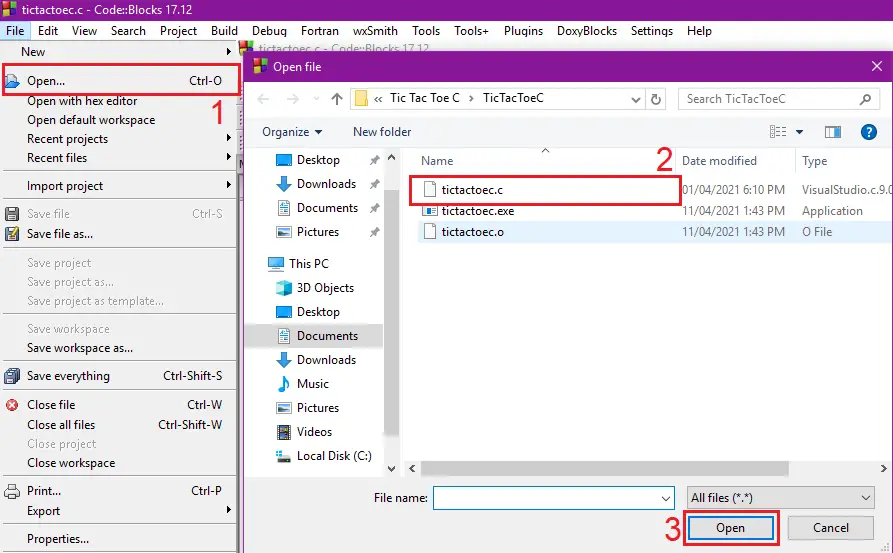• Step 5: Run Project

Fifth, run the project.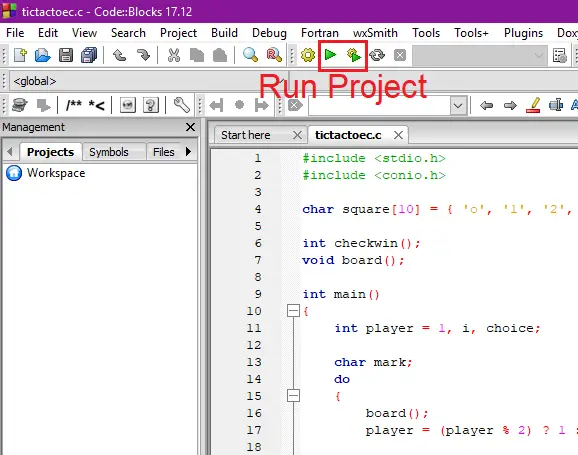• Step 6: The actual code.

#### The Code Given Below is the Actual Code of System

```#include <stdio.h>
#include <conio.h>

char square = { 'o', '1', '2', '3', '4', '5', '6', '7', '8', '9' };

int checkwin();
void board();

int main()
{
int player = 1, i, choice;

char mark;
do
{
board();
player = (player % 2) ? 1 : 2;

printf("   Player %d, enter a number:  ", player);
scanf("%d", &choice);

mark = (player == 1) ? 'X' : 'O';

if (choice == 1 && square == '1')
square = mark;

else if (choice == 2 && square == '2')
square = mark;

else if (choice == 3 && square == '3')
square = mark;

else if (choice == 4 && square == '4')
square = mark;

else if (choice == 5 && square == '5')
square = mark;

else if (choice == 6 && square == '6')
square = mark;

else if (choice == 7 && square == '7')
square = mark;

else if (choice == 8 && square == '8')
square = mark;

else if (choice == 9 && square == '9')
square = mark;

else
{
printf("   Invalid move ");

player--;
getch();
}
i = checkwin();

player++;
}while (i ==  - 1);

board();

if (i == 1)
printf("   ==>\aPlayer %d win ", --player);
else
printf("   ==>\aGame draw");

getch();

return 0;
}

int checkwin()
{
if (square == square && square == square)
return 1;

else if (square == square && square == square)
return 1;

else if (square == square && square == square)
return 1;

else if (square == square && square == square)
return 1;

else if (square == square && square == square)
return 1;

else if (square == square && square == square)
return 1;

else if (square == square && square == square)
return 1;

else if (square == square && square == square)
return 1;

else if (square != '1' && square != '2' && square != '3' &&
square != '4' && square != '5' && square != '6' && square
!= '7' && square != '8' && square != '9')

return 0;
else
return  - 1;
}

/*******************************************************************
FUNCTION TO DRAW BOARD OF TIC TAC TOE WITH PLAYERS MARK
********************************************************************/

void board()
{
system("cls");
printf("\n\n   \xcd\xcd\xcd\xcd\xcd\xcd\xcd\xcd\xcd\xcd\xcd\xcd\xcd\xcd\xcd\xcd\xcd\xcd\xcd\xcd\xcd\xcd\xcd\xcd\xcd\xcd\xcd\xcd\xcd\n");
printf("\t   Tic Tac Toe");
printf("\n   \xcd\xcd\xcd\xcd\xcd\xcd\xcd\xcd\xcd\xcd\xcd\xcd\xcd\xcd\xcd\xcd\xcd\xcd\xcd\xcd\xcd\xcd\xcd\xcd\xcd\xcd\xcd\xcd\xcd\n\n");

printf("   Player 1 (X)  -  Player 2 (O)\n\n");

printf("   \xcd\xcd\xcd\xcd\xcd\xcd\xcd\xcd\xcd\xcd\xcd\xcd\xcd\xcd\xcd\xcd\xcd\xcd\xcd\xcd\xcd\xcd\xcd\xcd\xcd\xcd\xcd\xcd\xcd\n");
printf("\t|     |     |     |\n");
printf("\t|  %c  |  %c  |  %c  |\n", square, square, square);

printf("\t|_____|_____|_____|\n");
printf("\t|     |     |     |\n");

printf("\t|  %c  |  %c  |  %c  |\n", square, square, square);

printf("\t|_____|_____|_____|\n");
printf("\t|     |     |     |\n");

printf("\t|  %c  |  %c  |  %c  |\n", square, square, square);

printf("\t|     |     |     |\n");
printf("   \xcd\xcd\xcd\xcd\xcd\xcd\xcd\xcd\xcd\xcd\xcd\xcd\xcd\xcd\xcd\xcd\xcd\xcd\xcd\xcd\xcd\xcd\xcd\xcd\xcd\xcd\xcd\xcd\xcd\n\n");
}
```